# Meet me up in space!

Rather than closing the distance, however, the target seemed to move down and away in defiance of everyday intuition...

David Austin
Grand Valley State University

Complex space missions rely on the ability to bring two spacecraft together, a procedure called orbital rendezvous. A spacecraft docking at the International Space Station is a typical example.

Historically, rendezvous was a vital component of the Apollo lunar missions. While three astronauts traveled to the moon in a single craft, the command module, two of them descended to the lunar surface in a second craft, the lunar excursion module (LEM). A successful mission required the ascending LEM to rendezvous with the command module before returning to Earth.Gemini 4 Capsule at the Smithsonian National Air and Space Museum.

The first attempt at orbital rendezvous was one component of the Gemini IV mission in 1965 when the pilot James McDivitt tried to bring his Gemini capsule close to the spent booster that lifted them into orbit. Upon first seeing the booster at an estimated 120 meters, McDivitt aimed the capsule at the booster and thrusted toward it. Rather than closing the distance, however, the target seemed to move down and away in defiance of everyday intuition. He repeated this procedure several times with similar results.

NASA engineer André Meyer later recalled, "There is a good explanation [for] what went wrong with rendezvous." Mission planners "just didn't understand or reason out the orbital mechanics involved" due to competing mission priorities. That's what we're going to do here. This column will explain the relative motion of two spacecraft flying closely in Earth orbit and why that motion behaves in such a counter-intuitive way.

Science fiction films often depict spacecrafts that continually alter their trajectories by firing their engines. In the real world, however, fuel is a scarce resource due to the energy required to lift the fuel into space. As a result, spacecraft spend most of their time coasting and only apply short bursts of thrust at opportune moments to adjust their trajectory. This means that a spacecraft operating near, say, Earth or the Moon will spend most of its time traveling in an elliptical orbit, as dictated by Kepler's second law.

We therefore imagine a target spacecraft $T$ traveling in a circular orbit, and an interceptor spacecraft $I$ attempting to rendezvous with the target and traveling in another elliptical orbit. It's not essential that the target's orbit be circular, but it's a convenient assumption for our purposes. The object of orbital rendezvous is to guide the interceptor so that it comes together with the target.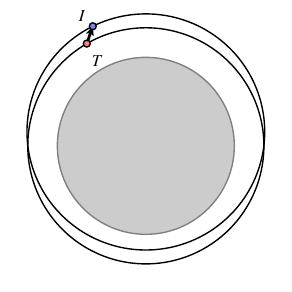### Equations of relative motion

The interceptor perceives the target as a fixed point that it wants to move toward. For this reason, we are interested in describing the motion of the interceptor in a coordinate system that rotates with the target, which means we want to understand the evolution of the vector joining the spacecrafts.

As a note to the reader, we'll walk through some calculations that explain the evolution of this vector and eventually find a rather elegant description. I'd like to give some intuition for what controls the motion, but I won't give every detail and trust that the reader can fill in as much or as little as they wish. This exposition follows a 1962 technical report by NASA engineer Donald Mueller and a more recent survey by Bradley Carroll. Apollo 11 astronaut Edwin "Buzz" Aldrin's M.I.T. Ph.D. thesis covers similar ground.

We'll denote the interceptor's location by $I$ and the target's by $T$. The vector ${\mathbf r}$ measures the displacement of the interceptor from the target. The vectors $\mathbf{R^*}$ and $\mathbf {r^*}$ measure the displacement of the target and interceptor from Earth's center.Since our goal is to understand the motion of the interceptor $I$ relative to the target $T$, we will focus our attention on ${\mathbf r}$. Moreover, we will consider a coordinate system that rotates with the target $T$ as shown below.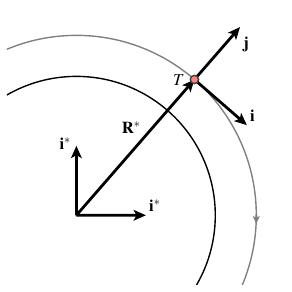For convenience, we will assume that the target is moving in a circular orbit of radius $R_0$ in the $xy$-plane. We see that ${\mathbf i}$ is a unit vector pointing in the direction of the target's motion, ${\mathbf j}$ points radially from the center of Earth, and ${\mathbf k}$ is a unit vector pointing out of the page.

The vectors ${\mathbf i}^*$, ${\mathbf j}^*$, and ${\mathbf k}^* = {\mathbf k}$ are fixed. In general, quantities denoted with a $*$ are with respect to this fixed, Earth-centered coordinate system and quantities without a $*$ are with respect to the rotating, target-centered coordinate system.

We can describe $${\mathbf R^*} = R_0\left[\cos(\omega_0T){\mathbf i}^* -\sin(\omega_0t){\mathbf j}^*\right],$$ where $\omega_0$ is the angular velocity of the target. Differentiating twice gives the acceleration $$\frac{d^2}{dt^2}{\mathbf R}^* = R_0\omega_0^2\left[-\cos(\omega_0T){\mathbf i}^* +\sin(\omega_0t){\mathbf j}^*\right].$$

Since gravity is the only force acting on the target, the magnitude of the acceleration vector is $GM/R_0^2$, where $G$ is the gravitational constant and $M$ is Earth's mass. This tells us that $R_0\omega_0^2 = GM/R_0^2$ so we have $$\omega_0=\sqrt{\frac{GM}{R_0^3}}.$$ Notice that the angular velocity decreases as the target's altitude increases.

This is really an expression of Kepler's Third Law for circular orbits. If $P$ is the period of the orbit, then $\omega_0P = 2\pi$, which says that $$P^2 = \frac{4\pi^2}{\omega_0^2} = \frac{4\pi^2}{GM}R_0^3$$ so that the square of the period is proportional to the cube of the radius.

We would like to relate rates of change measured in the fixed, Earth-centered coordinate system to those measured in the rotating, target-centered system. In particular, we are interested in the derivatives $$\frac{d^*}{dt}{\mathbf r},\hspace{24pt} \frac{d}{dt}{\mathbf r},$$ the first of which measures the rate of change of ${\mathbf r}$ in the fixed coordinate system while the second measures the rate of change in the rotating coordinate system.

Writing $${\mathbf r} = x{\mathbf i} + y{\mathbf j} + z{\mathbf k}$$ shows us that $$\frac{d^*}{dt}{\mathbf r} = \left(\frac{d}{dt}x\right){\mathbf i} + \left(\frac{d}{dt}y\right){\mathbf j} + \left(\frac{d}{dt}z\right){\mathbf k} + x\frac{d}{dt}{\mathbf i} + y\frac{d}{dt}{\mathbf j}.$$

Notice that there are some additional terms that come from differentiating the coordinate vectors. Introducing the angular velocity vector ${\mathbf \omega} = -\omega_0{\mathbf k}$ allows us to see, with a little calculation, that $$\frac{d^*}{dt}{\mathbf r} = \frac{d}{dt}{\mathbf r} + \omega\times{\mathbf r}.$$

After differentiating again, one can show that $$\frac{d^2}{dt^2}{\mathbf r} = \frac{{d^*}^2}{dt^2}{\mathbf r} - \omega\times(\omega\times{\mathbf r}) - 2\omega\times\frac{d}{dt}{\mathbf r}.$$

Remember that ${\mathbf r} = {\mathbf r}^* - {\mathbf R}^*$ so that $$\frac{{d^*}^2}{dt^2}{\mathbf r} = \frac{{d^*}^2}{dt^2}{\mathbf r}^* - \frac{{d^*}^2}{dt^2}{\mathbf R}^* = -\frac{GM}{|{\mathbf r}^*|^3}{\mathbf r}^* +\frac{GM}{|{\mathbf R}^*|^3}{\mathbf R}^*.$$ Since the target is moving in a circular orbit of radius $R_0$, we see that ${\mathbf R}^* = R_0{\mathbf j}$. Also, because ${\mathbf r}^* = {\mathbf R}^* + {\mathbf r}$, we have $${\mathbf r}^* = x{\mathbf i} + (R_0+y){\mathbf j} + z{\mathbf k}.$$

Since we are interested in guiding the interceptor to rendezvous with the target, we will assume that the distance between the target and interceptor is much less than the radius of the target's orbit. For instance, ${\mathbf r} = x{\mathbf i} + y{\mathbf j} + z{\mathbf k}$ may represent a length of a few kilometers, while ${\mathbf R}^* = R_0{\mathbf j}$ represents a length of several thousands of kilometers.

This leads us to approximate $|{\mathbf r}^*| \approx R_0 + y$ and to apply the linear approximation $$\frac{1}{|{\mathbf r}^*|^3} \approx \frac{1}{R_0^3} -\frac{3y}{R_0^4}.$$ With a little algebra, we can write the components of the vector $\frac{d^2}{dt^2}{\mathbf r}$ as

\begin{aligned} \frac{d^2x}{dt^2} & = -2\omega_0 \frac{dy}{dt}, \\ \frac{d^2y}{dt^2} & = 3\omega_0^2y + 2\omega_0\frac{dx}{dt}, \\ \frac{d^2z}{dt^2} & = -\omega_0^2z. \end{aligned}

These are known as the Hill equations that describe the interceptor's path in the rotating coordinate frame, and we will see how to find explicit solutions to them.

### Ellipses everywhere

The equation for the $z$-coordinate is a simple harmonic oscillator whose solution is $$z(t) = A\cos(\omega_0t) + B\sin(\omega_0t).$$

Remember that the $z$-axis is fixed in both coordinate systems. This form for $z(t)$ simply expresses the fact that in the fixed, Earth-centric coordinate system, the interceptor is traveling in an elliptical orbit about Earth's center. More specifically, this expression describes the motion of the interceptor out of the plane of the target's orbit. To achieve orbital rendezvous, we need to align the plane of the interceptor with that of the target. In practice, this incurs a considerable cost in fuel so care is taken to launch the interceptor in an orbital plane that's close to that of the target.

We will assume that the interceptor has performed such a maneuver so that its orbital plane agrees with that of the target. In other words, we'll assume that $z(t) = 0$ constantly and focus on the other two equations:

\begin{aligned} \frac{d^2x}{dt^2} & = -2\omega_0 \frac{dy}{dt}, \\ \frac{d^2y}{dt^2} & = 3\omega_0^2y + 2\omega_0\frac{dx}{dt}. \end{aligned}

We can develop some intuition by thinking about these equations more carefully. Remember that gravity is the only force acting on the interceptor. These equations tell us, however, that in the rotating coordinate system, the interceptor behaves as if it were subjected to two forces per unit mass:

\begin{aligned} {\mathbf F}_{\mbox{Tid}} & = 3\omega_0^2y{\mathbf j}, \\ {\mathbf F}_{\mbox{Cor}} & = -2\omega_0\frac{dy}{dt}{\mathbf i}+2\omega_0\frac{dy}{dt}{\mathbf j} = -2\omega\times\frac{d}{dt}{\mathbf r}. \end{aligned}

The first ${\mathbf F}_{\mbox{Tid}}$, known as the tidal force, tends to push the interceptor away from the target's circular orbit and makes $y=0$ an unstable equilibrium.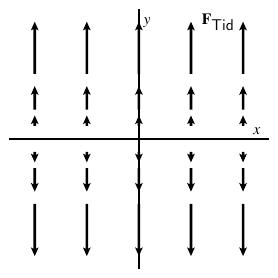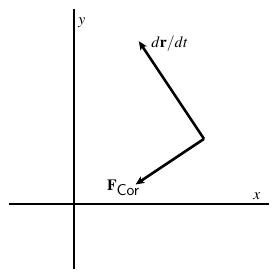The tidal force The Coriolis force

The second force, the Coriolis force, causes the interceptor to rotate counterclockwise as it moves in the rotating coordinate system.

Imagine what happens if we start just a little above the $x$-axis. The tidal force pushes us upward further away from the axis and causes us to pick up speed. The Coriolis force then begins to pull us to the left.

One simple solution to the equations of motion in the rotating frame, which can be verified directly from the equations, is given by

\begin{aligned} x(t) & = a\cos(\omega_0 t), \\ y(t) & = \frac a2\sin(\omega_0 t). \end{aligned}

Of course, we know by Kepler's Second Law that the interceptor's trajectory is elliptical in the fixed, Earth-centered frame. What's remarkable about this solution is that it says that the interceptor's path in the rotating frame is also elliptical.

The following figures illustrate this solution in both frames along with the positions at equal time intervals. It's worth taking a moment to study the relationship between them.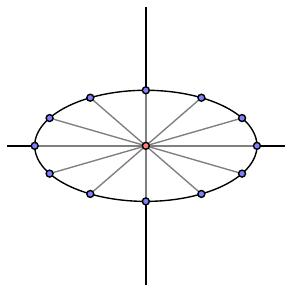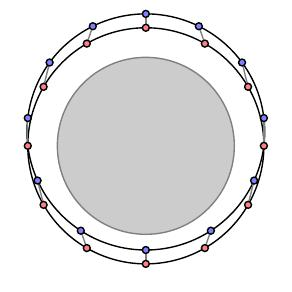In the rotating frame In the fixed frame

For this special solution, one can check that $$\frac{d^2}{dt^2}{\mathbf r} = -\omega_0^2{\mathbf r}.$$ This is the equation for a simple harmonic oscillator, the kind of motion observed by a mass on a spring. In other words, the interceptor moves as if it were connected to the target by a spring, which gives some insight into why orbital rendezvous is so counter-intuitive.

While this solution is certainly special, it shares many features with the general solution. Denoting the initial position by $(x_0, y_0)$ and the initial velocity by $(\dot{x}_0, \dot{y}_0)$, one can check that the general solution is
\begin{aligned} x(t) & = \left(6y_0 + \frac{4\dot{x}_0}{\omega_0}\right) \sin(\omega_0 t) + \frac{2\dot{y}_0}{\omega_0}\cos(\omega_0t) + \left[x_0 - \frac{2\dot{y}_0}{\omega_0} - (3\dot{x}_0 + 6\omega_0y_0)t\right], \\ y(t) & = -\left(3y_0 + \frac{2\dot{x}_0}{\omega_0}\right)\cos(\omega_0 t) + \frac{\dot{y}_0}{\omega_0}\sin(\omega_0t) + \left[4y_0 + \frac{2\dot{x}_0}{\omega_0}\right]. \end{aligned}

These expressions, known as the Clohessy-Wiltshire equations, initially look complicated, but a little study reveals a suprising simplicity. Defining

\begin{aligned} C & = 3y_0 + \frac{2\dot{x}_0}{\omega_0}, \\ D & = \frac{\dot{y}_0}{\omega_0}, \\ x_c & = x_0 - \frac{2\dot{y}_0}{\omega_0} - (3\dot{x}_0 + 6\omega_0y_0)t, \\ y_c & = 4y_0 + \frac{2\dot{x}_0}{\omega_0}, \end{aligned} enables us to write \begin{aligned} x(t) = 2C\sin(\omega_0 t) + 2D\cos(\omega_0 t) + x_c, \\ y(t) = -C\cos(\omega_0 t) + D\sin(\omega_0 t) + y_c. \end{aligned}

This shows that the interceptor, once again, is traveling in an elliptical path centered about the point $(x_c, y_c)$. In fact, the semi-major axis $a$ and semi-minor axis $b$ of the ellipse are

\begin{aligned} a & = 2\sqrt{C^2+D^2},\\ b & = a/2, \end{aligned}

which shows that the eccentricity of the path is always $\sqrt{3}/2$.

Notice, however, that the point $(x_c, y_c)$ is moving since $x_c$ has velocity $$v_{\mbox{drift}} = - (3\dot{x}_0 + 6\omega_0y_0)t = -\frac32\omega_0 y_c.$$ This is important because it says that the center of the ellipse drifts left if $y_c \gt 0$ and drifts right if $y_c \lt 0$.

### Parking orbits

Here are some special orbits that Mueller calls parking orbits.Remember that the orbit is determined by its initial conditions $(x_0,y_0)$ and $(\dot{x}_0,\dot{y}_0)$. With the right choice of initial conditions, we can arrange that $C=D=0$ so that the ellipse collapses to a point. Mueller's type I parking orbit has the interceptor in a circular orbit, as seen in the fixed frame, at the same altitude as the target. In the rotating frame, the interceptor is not moving relative to the target.A type III parking orbit has the interceptor again in a circular orbit but at a different altitude than the target. Since the angular velocity decreases with the altitude, the interceptor appears to move horizontally in the rotating frame. The Clohessy-Wiltshire equations explain this by noting that the center of the ellipse moves left when $y_c\gt0$ and right when $y_c\lt0$.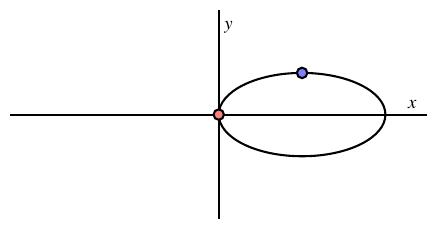Things get a little more interesting if we allow $C$ and $D$ to be nonzero so that the interceptor moves on an elliptical trajectory in the rotating frame. A type II parking orbit has $y_c = 0$ so that the center of the ellipse is centered on the $x$-axis. In this case, the center does not move so the elliptical orbit is stationary. The special solution we looked at earlier is an example. The orbit depicted here shows how an astronaut could retrieve a tool accidentally dropped by just waiting one orbital period for its return.A type IV orbit has the interceptor traveling an elliptical orbit whose center is drifting. The orbit shown has $y_c \gt 0$ so the center of the ellipse is moving to the left.

With any solution, one can check that the interceptor moves as if connected by a spring to the moving center of the ellipse, which again speaks to the counter-intuitive nature of the motion.

These orbits explain the behavior of Gemini IV, which we described in the introduction. Imagine that the interceptor is initially in a type I parking orbit, traveling in the same circular orbit as the target and 500 meters away.McDivitt aimed at the target and fired Gemini's thruster, which would make $\dot{x}_0 \gt 0$ and move the craft into the type IV orbit shown here. This explains why the target paradoxically appeared to move down and away from the capsule.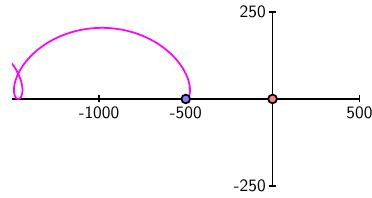One scenario Carroll considers has an astronaut stranded outside the ISS at an initial position of $(100,100)$, which is 100 meters in front of the space station and 100 meters above. Applying a momentary thrust to create a velocity of 1 meter per second aimed directly at the space station leads to a type IV orbit that badly misses the station.So how can we put the interceptor on a path to dock with the target? We'll assume the interceptor is at an initial position $(x_0, y_0)$ and choose a time $T$ at which we'd like to dock. Setting $x(T) = 0$ and $y(T) = 0$ gives the equations

\begin{aligned} x(T) = 0 & = \left(6y_0 + \frac{4\dot{x}_0}{\omega_0}\right) \sin(\omega_0 T) + \frac{2\dot{y}_0}{\omega_0}\cos(\omega_0T) + \left[x_0 - \frac{2\dot{y}_0}{\omega_0} - (3\dot{x}_0 + 6\omega_0y_0)T\right], \\ y(T) = 0 & = -\left(3y_0 + \frac{2\dot{x}_0}{\omega_0}\right)\cos(\omega_0 T) + \frac{\dot{y}_0}{\omega_0}\sin(\omega_0T) + \left[4y_0 + \frac{2\dot{x}_0}{\omega_0}\right]. \end{aligned}

Once again, these equations appear complicated, but notice that we know everything except the components of the initial velocity. This means that these are two linear equations for the two components of the unknown initial velocity $(\dot{x}_0, \dot{y}_0)$. Once we have found those components, the interceptor applies a momentary thrust to change its velocity to $(\dot{x}_0, \dot{y}_0)$ bringing it the target's position at time $T$.

Carroll's paper shows how to apply this idea to reproduce the rendezvous technique used in the Apollo 11 mission as Neil Armstrong and Buzz Aldrin returned from the lunar surface to dock with Michael Collins in the command module.

### Summary

NASA learned from Gemini IV's failure to rendezvous with the booster target, and several months later, the Gemini VI mission successfully navigated to within 130 feet of Gemini VII. The pilot of Gemini VI, Walter Schirra, later explained that the difficulty was getting to that distance but that completing docking from there is relatively straightforward. Carroll's paper examines that claim and confirms that line-of-sight flying is effective within about 100 feet of the target.The International Space Station in 2021.

In the past, spacecraft docking with the International Space Station would fly in formation with the station, which would grab the craft with the Canadarm2 and manually complete the docking procedure. Recently, however, SpaceX has automated the docking process so that software guides the craft rather than astronauts who are not involved at all.

Would you like to try to dock with the ISS? This SpaceX simulator gives you the chance.